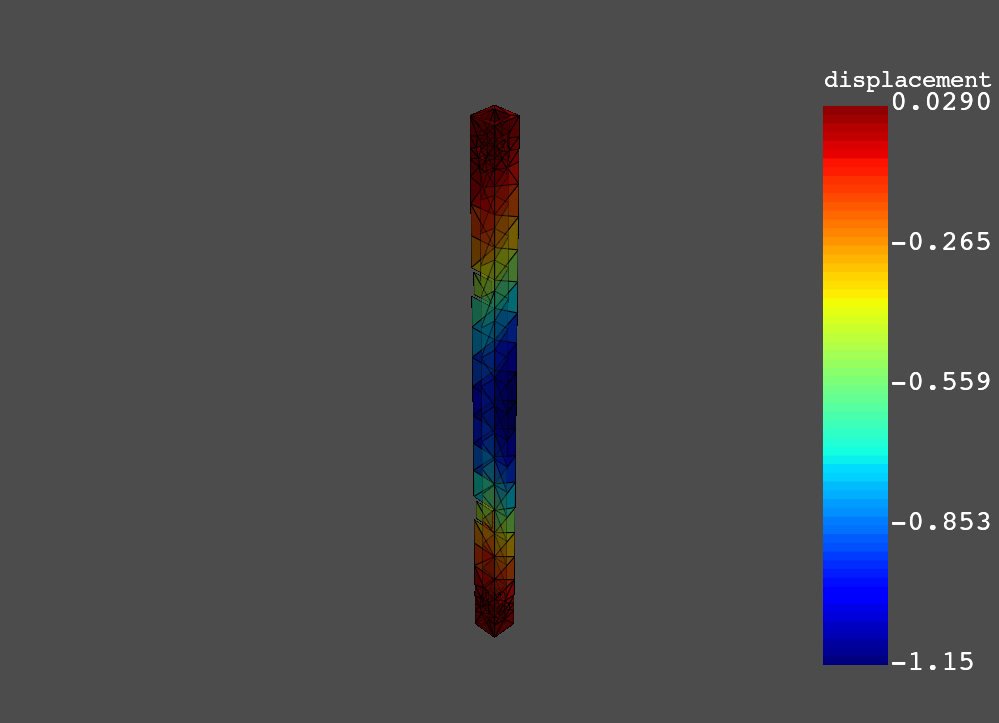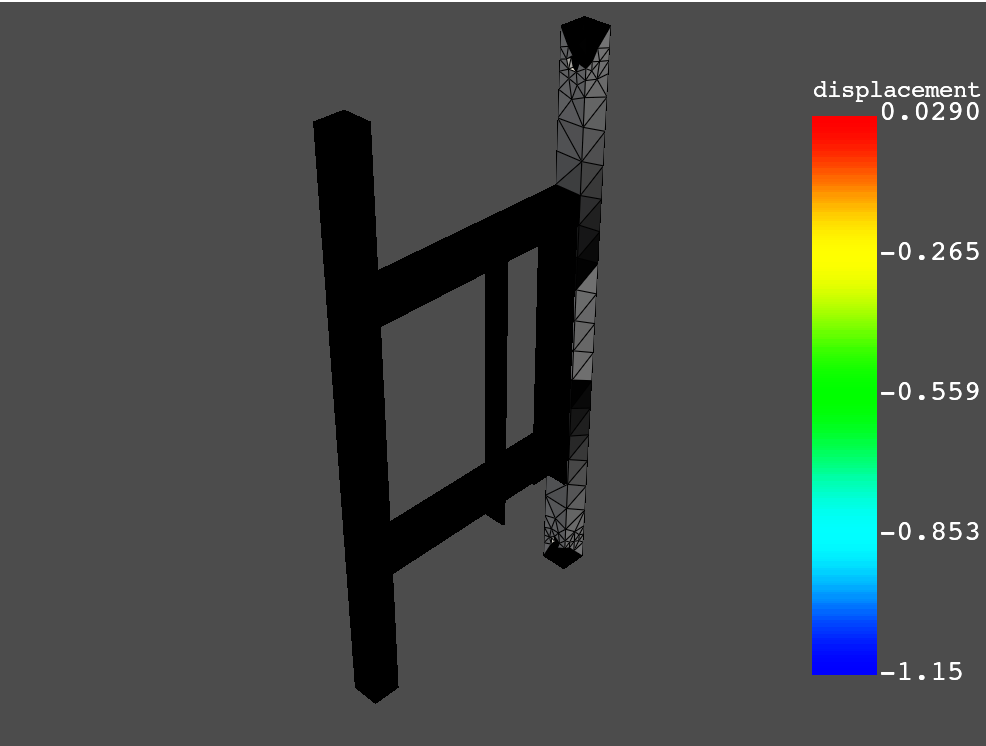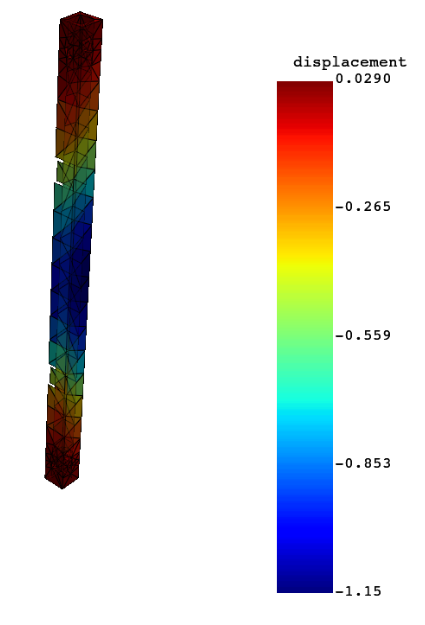# How can I plot in pydpf-post results on a mesh part linked to a named selection?Member, Employee Posts: 55'Extract results on named selections - Modal Simulation' example shows how to plot results on a specific named selection. Results are only shown on that named selection, but the rest of the structure is kept grey. Is there any way to only plot the mesh which is linked to the named selection and not the whole structure?

Tagged:

•Member, Employee Posts: 55Having a look at pydpf-post manual, we can see that simulation.plot has only mesh=true or mesh=false, but it does not allow to link to a specific part of the mesh. What it can be done is to use plot contour and its pyvista options, for instance opacity.
Here the code to plot on BAR_1 named selection from the example commented before:

```from ansys.dpf import post
from ansys.dpf.post import examples

disp = solution.displacement()
disp_bar_1 = solution.displacement(named_selection='BAR_1')
dxb1 = disp_bar_1.x

all_elem = solution.mesh.elements.scoping.ids
elem_ns = solution.mesh.named_selection('BAR_1').ids

opacities = []

for i in all_elem:
if i in elem_ns:
opacities.append(1.0)
else:
opacities.append(0.0)

pl = dxb1.plot_contour("time", , opacity=opacities)
```

The result got is the following:Another option would be using pydpf-core to generate a mesh from scoping and plot the desired result on it.

•Member Posts: 4When I copy paste the code I get this:Any idea what might be wrong?

•Member, Employee Posts: 55edited September 26

Hello @Nathanvanthof ,
It seems something related to pyvista. An alternative to the workaround that I proposed is to apply a opacity of 0.0 to all the elements with no result, which gives same results for me, as you can see in the screenshot below. These would be the code:

```from ansys.dpf import post
from ansys.dpf.post import examples

disp = solution.displacement()
disp_on_named_selection = solution.displacement(named_selection='BAR_1')
dx = disp_on_named_selection.x

all_elem = solution.mesh.elements.scoping.ids
elem_ns = solution.mesh.named_selection('BAR_1').ids

pl = dx.plot_contour("time", , nan_opacity = 0.0)
```If you are still having issues, I would play with options given in pyvista.plot and pv.Plotter.add_mesh.
Regards, Javier.

•Member Posts: 4This works, thank you so much!